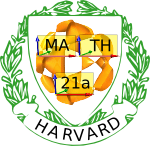6760, Math 21a, Fall 2009
Exhibits page Math 21a 2009, Multivariable Calculus
Stokes becomes Green
Office: SciCtr 434

 Stokes theorem states that ```intS curl F . dS = intC F . dr ``` If we rewrite the left hand side in terms of a parametrization r(u,v) from a region D in the u-v plane, we get intD curl(F) . (ru x rv) du dv If we rewrite the right hand side in terms of this parametrization, we get, letting B be the boundary of D ``` intB (F . ru, F . rv) . dr ``` (Check this! it amounts to the chain rule). By Green's theorem (applied in the u-v plane) this is ```intD d/du (F . rv) - d/dv (F . ru) du dv ``` Thus if we can show that ```curl(F) . (ru x rv) = d/du (F . rv) - d/dv (F . ru) ``` we'll have shown that Stokes theorem follows from Green's theorem. This can be done using vector triple product identities as follows: Lets pretend first the vectors ru and rv are constant in u and v, so that only derivatives of F matter: by identities which can be found in 9.4 of the book and neglecting further derivatives of ru because ru and rv are constant: ```(nabla x F) . (ru x rv) = ((nabla x F) x ru) . rv = ((ru . nabla) F - nabla (F . ru)) . rv = (ru . nabla)(F . rv) - (rv . nabla)(F . ru) = d/du (F . rv) - d/dv (F . ru) (*) = dF/du . rv - dF/dv . ru (**) ``` Now lets stop pretending ru and rv are constant. We'd really like the original expression (*), but with ru and rv not constant, so let's try expanding (*) via the chain rule. We get: ```dF/du . rv + F . rvu - dF/dv . ru - F . ruv. ``` Thankfully, two terms cancel by Clairaut's theorem, and the two expressions (*) and (**) are equal, even if ru and rv are allow to vary. If P(u,v) = F . ru and Q(u,v) = F . rv,, we see that the line integral around the boundary, using the parametrization, is precisely the line integral with respect to the vector field (P,Q). Stokes Theorem is reduced to Green's Theorem.